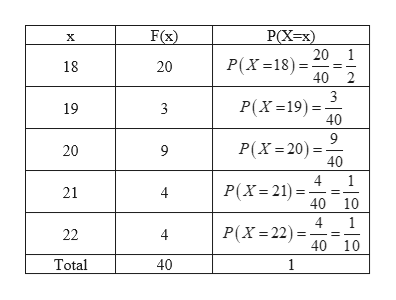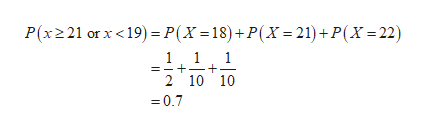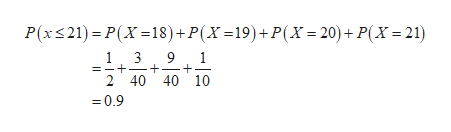xx18181919202021212222F(x)F(x)202033994444Let xx be the ages of students in a class. Given the frequency distribution F(x)F(x) above, determine the following probabilities:(a) P(x≥21 or x<19)=P(x≥21 or x<19)= equation editorEquation Editor (b) P(x≤21)=P(x≤21)= equation editorEquation Editor (c) P(x<22)=P(x<22)=

Question
 xx 1818 1919 2020 2121 2222 F(x)F(x) 2020 33 99 44 44

Let xx be the ages of students in a class. Given the frequency distribution F(x)F(x) above, determine the following probabilities:

(a) P(x≥21 or x<19)=P(x≥21 or x<19)=

equation editor
Equation Editor

(b) P(x≤21)=P(x≤21)=

equation editor
Equation Editor

(c) P(x<22)=P(x<22)=

Step 1

From the given frequency distribution, the corresponding probabilities are given in the below table.help_outlineImage TranscriptioncloseF(x) РX-x) 20 1 Р(X -18) - 18 20 40 2 3 Р(х -19) - 40 19 3 9 P(X 20 40 20 9 4 1 P(X 21 21 40 10 4 P(X 22) 22 4 40 10 Total 40 1 fullscreen
Step 2

a).

The required probability can be obtained as follows.help_outlineImage TranscriptioncloseP(x221 or x <19)P(X =18)+P(X 21)+P(X=22) 11 1 2 10 10 =0.7 fullscreen
Step 3

b).

The required probability can b...help_outlineImage TranscriptioncloseP(x21) P(X 18)+P(X =19)+P(X=20)+ P(X 21) 9 1 1 3 2 40 40 10 =0.9 fullscreen

Want to see the full answer?

See Solution

Want to see this answer and more?

Our solutions are written by experts, many with advanced degrees, and available 24/7

See Solution
Tagged in

Other